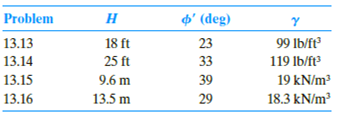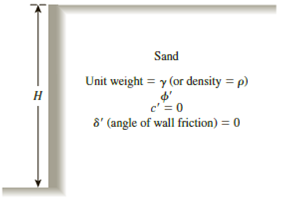Chapter 13, Problem 13.16PPrinciples of Geotechnical Enginee...

9th Edition
Braja M. Das + 1 other
ISBN: 9781305970939

Solutions

Chapter
SectionPrinciples of Geotechnical Enginee...

9th Edition
Braja M. Das + 1 other
ISBN: 9781305970939
Textbook Problem

13.13 through 13.16 Assume that the retaining wall shown in Figure 13.39 is frictionless. For each problem, determine the Rankine passive force per unit length of the wall, the variation of active earth pressure with depth, and the location of the resultant.Figure 13.39

To determine

Find the Rankine passive force σp(z=H) per unit length of the wall, the variation of active earth pressure Pa with depth, and the location z¯ of the resultant measured from the bottom of the wall.

Explanation

Given information:

The height (H) of the retaining wall is 13.5 m

The soil friction angle ϕ is 29°.

The angle of wall friction δ is 0.

The unit weight γ of the soil is 18.3kN/m3.

Calculation:

Calculate the passive earth pressure coefficient (Kp) using the relation.

Kp=tan2(45+ϕ2)

Substitute 29° for ϕ.

Ka=tan2(45+29°2)=tan2(45+14.5)=tan2(59.5)=2.882

Calculate Rankine passive force σp(z=H) per unit length of the wall using the relation.

σp(z=H)=KpγH

Substitute 2.882 for Kp, 18.3kN/m3 for γ, and 13.5 m for H.

σp(z=H)=2

Still sussing out bartleby?

Check out a sample textbook solution.

See a sample solution

The Solution to Your Study Problems

Bartleby provides explanations to thousands of textbook problems written by our experts, many with advanced degrees!

Get Started

Calculate the second moment of area of a 4 in. diameter shaft about the xx and yy axes, as shown.

Engineering Fundamentals: An Introduction to Engineering (MindTap Course List)

Define the term “computer network.”

Fundamentals of Information Systems

Describe the strategy of acceptance.

Management Of Information Security

Convert 5 megawatts of power into BTU/hr, ft-lbs/s, and kJ/hr.

Fundamentals of Chemical Engineering Thermodynamics (MindTap Course List)

Define an object, and provide three examples.

Systems Analysis and Design (Shelly Cashman Series) (MindTap Course List)

Differentiate among voice, text, picture, and video messages.

Enhanced Discovering Computers 2017 (Shelly Cashman Series) (MindTap Course List)